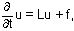18.156 | Spring 2004 | Graduate

# Differential Analysis

## Syllabus

### Course Meeting Times

Lectures: 2 sessions / week, 1.5 hours / session

### Description

The main goal of this course is to give the students a solid foundation in the theory of elliptic and parabolic linear partial differential equations. We will begin with the theory of harmonic functions, and then study maximum principles for more general elliptic and parabolic equations. We will then discuss Schauder theory, that is, Hölder estimates for second derivatives of solutions to linear equations. If we have an equation of the form

Lu = f, orwhere L is a linear second order elliptic operator, then when does C^{alpha} regularity of f imply C^{2,alpha} regularity of u?

We will also discuss Sobolev spaces and the L^{p} theory for linear equations; the analogous question here is when does L^{p} regularity of f imply W^{2,p} regularity of u? We will then show some applications of this regularity theory of solutions to linear equations to the theory of nonlinear partial differential equations.

### Prerequisite

Differential Analysis (18.155)

### Textbook

#### Required Text

Jost, Jurgen. Partial Differential Equations. New York: Springer-Verlag, 2002. ISBN: 0387954287.

Gilbarg, David, and Neil Trudinger. Elliptic Partial Differential Equations of Second Order. 2nd ed. Berlin: Springer-Verlag, 1983.

The basis for the course grade is class attendance and turning in homework assignments.

## Course Info

Spring 2004
##### Learning Resource Types
Lecture Notes
Presentation Assignments